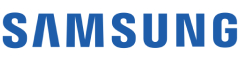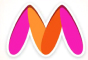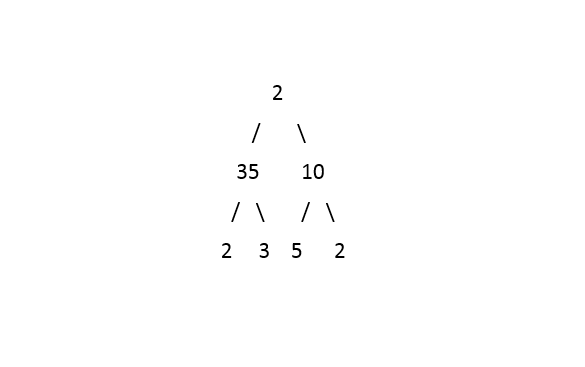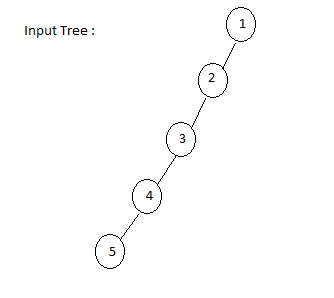New update is available. Click here to update.
Topics

# Right View

Moderate0/80
Average time to solve is 35m+36 more companies

## Problem statement

You have been given a Binary Tree of integers.

The right view of a Binary Tree is a set of nodes visible when the tree is viewed from the Right side and the nodes are printed from top to bottom order.

Detailed explanation ( Input/output format, Notes, Images )
Constraints:
``````1 <= T <= 100
1 <= N <= 10^5
-10^9 <= data <= 10^9

Where 'N' is the number of nodes in the tree and 'data' is the value of a node in the given tree.

Time Limit: 1 sec
``````
Sample Input 1 :
``````1
2 35 10 2 3 5 2 -1 -1 -1 -1 -1 -1 -1 -1
``````
Sample Output 1 :
``````2 10 2
``````
Explanation of The Sample Input 1:``````The right view of the tree contains all the extreme-right elements in each level of the tree, including the head of the tree.
``````
Sample Input 2 :
``````1
1 2 -1 3 -1 4 -1 5 -1 -1 -1
``````
Sample Output 2 :
``````1 2 3 4 5
``````
Explanation of The Sample Input 2 :Console